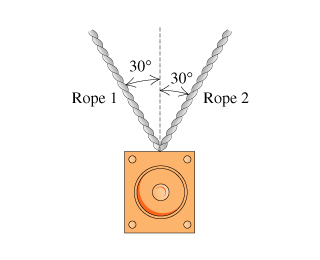# Problem: A 20-kg loudspeaker is suspended 2.0 m below the ceiling by two ropes that are each 30° from vertical.A) Find the value of T, the magnitude of the tension in either of the ropes.Express your answer in newtons.T=B) Suppose that the loudspeaker in the problem had a mass of 500 kg and the ropes hung 20° from the vertical. Into which of the following intervals would you expect T to fall? You do not have to calculate Texactly to answer this question; just make an estimate.A. 500 to 1000 NB. 1000 to 2000 NC. 2000 to 4000 ND. 4000 to 8000 NE. 8000 to 16,000 N

###### FREE Expert Solution

In this problem, the forces must be resolved along the x and y directions then apply Newton's second law to determine the tension acting on the cables.

93% (256 ratings)###### Problem Details

A 20-kg loudspeaker is suspended 2.0 m below the ceiling by two ropes that are each 30° from vertical.A) Find the value of T, the magnitude of the tension in either of the ropes.

T=

B) Suppose that the loudspeaker in the problem had a mass of 500 kg and the ropes hung 20° from the vertical. Into which of the following intervals would you expect T to fall? You do not have to calculate Texactly to answer this question; just make an estimate.

A. 500 to 1000 N

B. 1000 to 2000 N

C. 2000 to 4000 N

D. 4000 to 8000 N

E. 8000 to 16,000 N